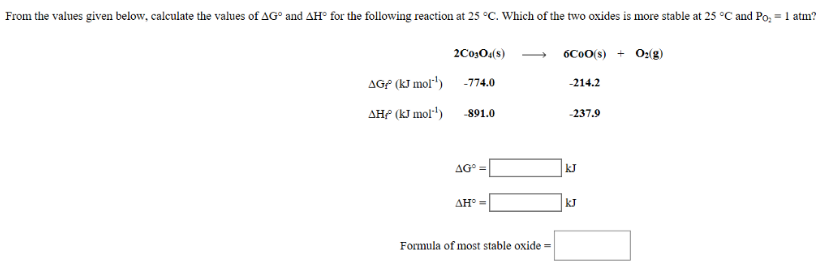# Problem: From the values given below, calculate the values of ΔG° and ΔH° for the following reaction at 25°C. Which of the two oxides is more stable at 25°C and PO2 = 1 atm? 2Co3O4 (s) → 6CoO (s) + O2 (g)

###### FREE Expert Solution
80% (454 ratings)###### Problem Details

From the values given below, calculate the values of ΔG° and ΔH° for the following reaction at 25°C. Which of the two oxides is more stable at 25°C and PO2 = 1 atm?

2Co3O(s) → 6CoO (s) + O2 (g)